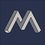# Sum of largest and smallest elements in a subset

## Question

In how many subsets of $\{ 1, 2, 3, 4, \ldots n\}$ is the sum of the largest element and the smallest element equal to $(n+1)$?

## Solution

I am able to deduce the following:

• If the smallest element is $1$, then the largest element has to be $n$. The rest of the $n-2$ elements can be "in" or "out", thus the total will be $2^{n-2}$
• Similarly, If the smallest element is $2$, then the largest element has to be $n-1$. And again, it will have $2^{n-4}$ such subsets
• $\ldots \ldots$

• If $n$ is odd the total subsets will be: $2^{n-2} + 2^{n-4} + \ldots + 2^{1}$
• If $n$ is even the total subsets will be: $2^{n-2} + 2^{n-4} + \ldots + 2^{0}$Note by Mahdi Raza
1 year, 1 month ago

This discussion board is a place to discuss our Daily Challenges and the math and science related to those challenges. Explanations are more than just a solution — they should explain the steps and thinking strategies that you used to obtain the solution. Comments should further the discussion of math and science.

When posting on Brilliant:

• Use the emojis to react to an explanation, whether you're congratulating a job well done , or just really confused .
• Ask specific questions about the challenge or the steps in somebody's explanation. Well-posed questions can add a lot to the discussion, but posting "I don't understand!" doesn't help anyone.
• Try to contribute something new to the discussion, whether it is an extension, generalization or other idea related to the challenge.

MarkdownAppears as
*italics* or _italics_ italics
**bold** or __bold__ bold
- bulleted- list
• bulleted
• list
1. numbered2. list
1. numbered
2. list
Note: you must add a full line of space before and after lists for them to show up correctly
paragraph 1paragraph 2

paragraph 1

paragraph 2

[example link](https://brilliant.org)example link
> This is a quote
This is a quote
    # I indented these lines
# 4 spaces, and now they show
# up as a code block.

print "hello world"
# I indented these lines
# 4 spaces, and now they show
# up as a code block.

print "hello world"
MathAppears as
Remember to wrap math in $$ ... $$ or $ ... $ to ensure proper formatting.
2 \times 3 $2 \times 3$
2^{34} $2^{34}$
a_{i-1} $a_{i-1}$
\frac{2}{3} $\frac{2}{3}$
\sqrt{2} $\sqrt{2}$
\sum_{i=1}^3 $\sum_{i=1}^3$
\sin \theta $\sin \theta$
\boxed{123} $\boxed{123}$

Sort by:

Can anyone suggest a cleaner and short-hand form for the above answer?

- 1 year, 1 month ago

This is good. Nothing to improve on.

- 1 year, 1 month ago

Ok.

- 1 year, 1 month ago

The best answer possible is given. You can also write it as: $\sum_{n\geq k \geq2:2|k}2^{n-k}$

- 1 year, 1 month ago

Hmm.. it is pretty compact but could be hard to understand. Thanks though!

- 1 year, 1 month ago

Pretty good!

- 1 year, 1 month ago

Thanks!

- 1 year, 1 month ago

No problem!

For any integer $x$ and any prime $p$, can

$\frac{x}{p}$

always be irreducible if $x$ isn't a factor of $p$?

Make a note on it if you have proof.

- 1 year, 1 month ago

If you know the definition of a prime number, this should be obvious to prove/disprove.

- 1 year, 1 month ago

Yes. And I think the question should say "x isn't a multiple of p" in which case the answer should obviously be a yes unless I am missing out something very trivial. Here is a simple deduction

Let $\frac{x}{p} = k$ where '$k$' is an integer.

For $k$ to be an integer, $x = pk$

That means $x$ has to obviously be a multiple of $p$.

- 1 year, 1 month ago

Great! You did see my comment to @Hamza Anushath?

- 1 year, 1 month ago